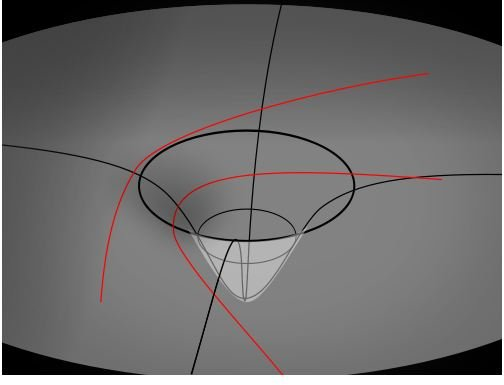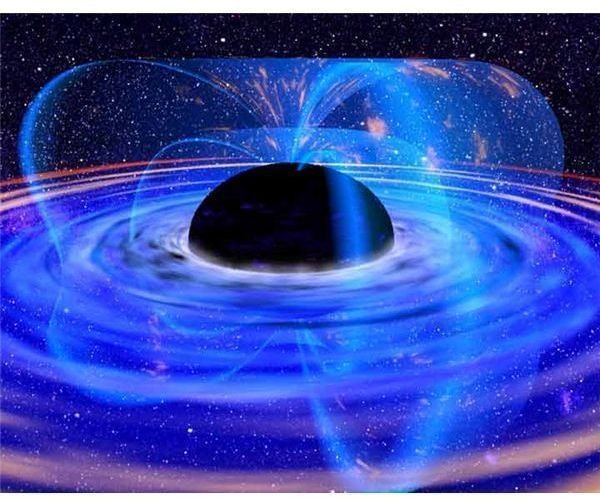# Gravity in Space: How Gravitational Force Interacts with the Cosmos

Page content

## Understanding Gravity

Gravity is a force of attraction. It is present on Earth and throughout the Universe. It is one of the four fundamental forces of nature. Gravity was defined by Sir Isaac Newton in his Principia and later by Einstein in his theory of General Relativity. Both explanations are different. Physicists use Einstein’s theory to explain the physics of large masses, whereas for the orbital motions of planets and trajectories of spacecrafts, Newton’s theory of gravity is used. Let us briefly look at both of these theories.

## Newton’s Universal Law of Gravitation

Newton’s universal law of gravitation states that every object in the Universe attracts another object with a force that is directly proportional to the product of their masses and inversely proportional to the distance between the objects. According to Newton, two objects in the Universe are attracting one another with a gravitational force that will increase if the distance between them is reduced and decrease if the distance between them is increased. This law was conventionally used and made certain predictions about planets that were not yet discovered, but were found as a consequence of the theory. In his theory of gravitation, Newton also introduced the gravitational constant (G) which is universal and has a value of 6.67 x 10-11 N (m/kg)2.

## Einstein’s Theory of General RelativityEinstein proposed a theory of gravity which had its starting point in the equivalence principle. Einstein’s take on the equivalence principle was that an observer on Earth is experiencing the same force of gravity as an observer in free-fall due to constant acceleration.

General relativity describes gravity as a curvature of space-time. In other words, objects in the fabric of space-time bend it so as to create gravity. Massive bodies like the Sun create a big bend in the fabric of space-time and other objects such as planets simply follow this curvature. The theory of relativity is comprised of a set of 10 equations aptly called the Einstein field equations. The equations are non-linear, differential equations and solutions to them have yielded astounding results which help us understand how gravity works in space. We will examine some of these solutions in an attempt to understand how gravitational force works in space.

## Schwarzschild Black HoleOne of the solutions of the Einstein field equations is the Schwarzschild black hole. A black hole has enormous gravity and such objects were thought to only exist in theory until they were discovered by scientists as a result of the Einstein field equations. Schwarzschild black holes have singularities, points at which objects cannot be defined. The Schwarzschild radial coordinate within the black hole becomes more like time and the time coordinate becomes more like space. The outer surface of the black hole is called the event horizon and gravity is so strong in this region that not even light can escape from it. The fabric of space-time is so curved that if one were to enter the black hole gravity would literally cause one to disintegrate, almost like a stretching effect, to subatomic particles. It has however been postulated that if one could escape the gravitational forces of a black hole they would find a portal to a remote region of the Universe or possibly even a parallel universe.

## Time Dilation

Gravitational time dilation is yet another one of the predictions of general relativity. With varying regions of gravitational potential, time dilates or passes slower or faster in different regions—a key element in making our GPS work. This prediction has been measured by atomic clocks and it is mind-bending suggesting that objects that are really massive will cause this effect. Objects traveling at relativistic speeds will also cause time dilation.

## In Conclusion

Understanding gravity is a complex thing to do. Scientists have only begun to understand the various effects of gravity in space and are only at the beginning stages of understanding this complex force. Other predictions of general relativity also exist such as gravitational lensing and the Kerr solution for rotating massive objects. Yet, what wonders gravity may bring to us in the future can only be someone’s guess.

## Credits

1. https://en.wikipedia.org/wiki/Schwarzschild_solution

4. https://www.ehow.com/how-does_4675197_gravity-work.html

Image Credits: WikimediaCommons/Dna-Dennis(https://commons.wikimedia.org/wiki/File:NewtonsLawOfUniversalGravitation.svg)/Cronholm144(https://commons.wikimedia.org/wiki/File:Geodesiques.svg)/NASA(https://commons.wikimedia.org/wiki/File:BlackHole.jpg)